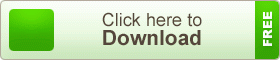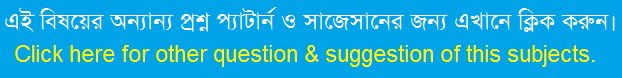# HSC Higher Mathematics 2nd Paper Note 7th Chapter Inverse Trigonometric Functions and Trigonometric Equations

HSC Higher Mathematics 2nd Paper Note 7th Chapter Inverse Trigonometric Functions and Trigonometric Equations. In mathematics, the inverse trigonometric functions (occasionally also called arcus functions, antitrigonometric functions or cyclometric functions are the inverse functions of the trigonometric functions (with suitably restricted domains). Specifically, they are the inverses of the sine, cosine, tangent, cotangent, secant, and cosecant functions, and are used to obtain an angle from any of the angle’s trigonometric ratios. Inverse trigonometric functions are widely used in engineering, navigation, physics, and geometry.

## HSC Higher Mathematics 2nd Paper Note 7th Chapter Inverse Trigonometric Functions and Trigonometric EquationsThere are several notations used for the inverse trigonometric functions. The most common convention is to name inverse trigonometric functions using an arc- prefix, e.g., arcsin(x), arccos(x), arctan(x), etc. This convention is used throughout the article. When measuring in radians, an angle of θ radians will correspond to an arc whose length is rθ, where r is the radius of the circle. Thus, in the unit circle, “the arc whose cosine is x” is the same as “the angle whose cosine is x”, because the length of the arc of the circle in radii is the same as the measurement of the angle in radians. Similarly, in computer programming languages the inverse trigonometric functions are usually called asin, acos, atan.

teachingbd24.com is such a website where you would get all kinds of necessary information regarding educational noes, suggestions and questions’ patterns of school, college, and madrasahs. Particularly you will get here special notes of physics that will be immensely useful to both students and teachers. The builder of the website is Mr. Md. Shah Jamal Who has been serving for 30 years as an Asst. Professor of BAF Shaheen College Dhaka. He expects that this website will meet up all the needs of Bengali version learners /students. He has requested both concerned students and teachers to spread this website home and abroad.